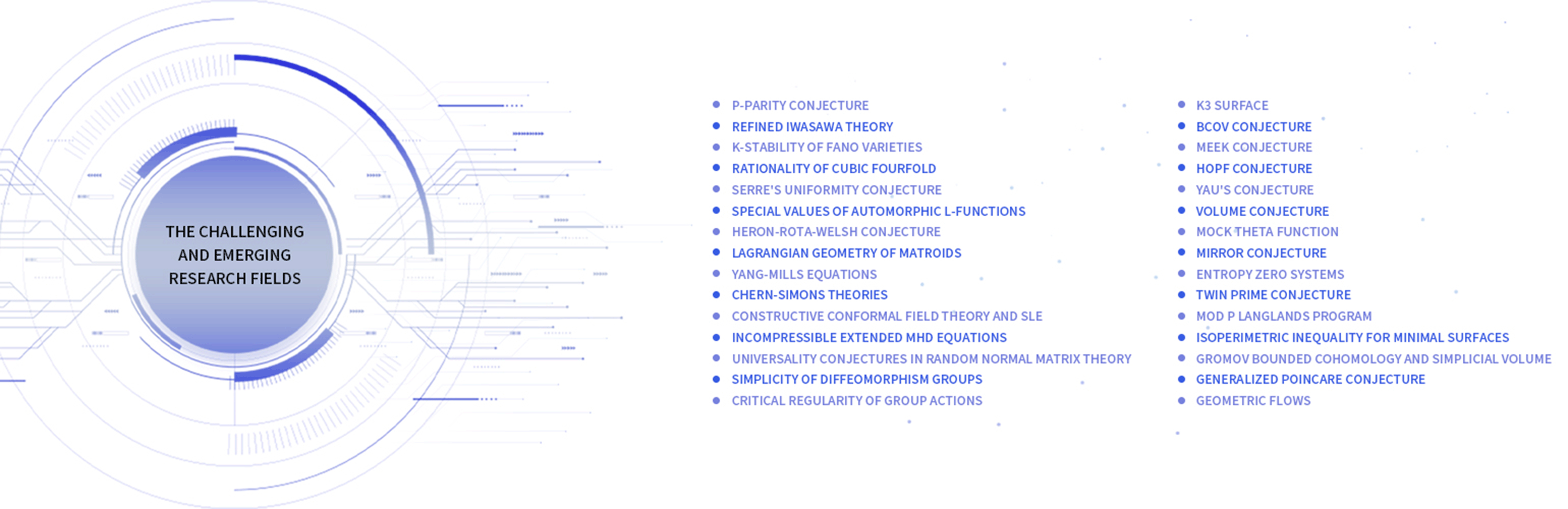Centers & Programs

# Introduction

Home Centers & Programs Mathematical Challenges Introduction

The Center for Mathematical Challenges (CMC) was established by the Korea Institute for Advanced Study (KIAS) to resolve mathematical challenges and present emerging strategies in new fields. The CMC also seeks to serve as a global hub in solving mathematical problems and in satisfying the intellectual curiosity of young and adventurous mathematicians around the world.

The CMC aspires to attain international leadership by focusing on well-known mathematical challenges and creating synergy as a national brand for South Korea. It will contribute to the enhancement of national competitiveness in basic science and technology, which will ultimately result in a positive impact on humanity.

* CMC is on the 3rd floor of Bld.8 of KIAS.

### Research Fields

The CMC deals with the problems in the challenging and emerging research fields;• Special Values of Automorphic L-Functions

• Lagrangian Eometry of Matroids

• Heron-Rota-Welsh Conjecture

• Serre’s Uniformity Conjecture

• Rationality of Cubic Fourfold

• K-stability of Fano Varieties

• Refined Iwasawa Theory

• P-Parity Conjecture

• yang-mills Equations

• Conformal Field Theory

• Chern-simons Theories

• Random Normal Matrix Theory

• Incompressible Extended MHD Equations

• Universality Conjecture for Random Normal Matrix

The Challenging Research Fields

• Isopeimetric Inequality for Minimal Surfaces

• Twin prime Conjecture

• Entropy Zero Systems

• Mock Theta Function

• Volume Conjecture

• Meek Conjecture

• Hopf Conjecture

• K3 Surface

• Yau’s Conjecture

• BCOV Conjecture

• Mirror Conjecture

• MOD P Lanlands Program

• Rigorization of Conformal Field Theory

• Gromov Bounded Cohomology and Simplicial volume

and more...